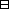## Group Theory and Linear Algebra

Last updated: 24 September 2014

## Lecture 24: $G/\text{ker} f\simeq \text{im} f$

Group homomorphisms are for comparing groups.

Let $G$ and $H$ be groups. A group homomorphism from $G$ to $H$ is a function $f:G\to H$ such that

 (a) If ${g}_{1},{g}_{2}\in G$ then $f\left({g}_{1}{g}_{2}\right)=f\left({g}_{1}\right)f\left({g}_{2}\right)\text{.}$

Let $f:G\to H$ be a group homomorphism. The kernel of $f$ is $ker f={g∈G | f(g)=1}.$ The image of $g$ is $im f= {f(g) | g∈G}.$

Let $f:G\to H$ be a group homomorphism. Then $\text{ker} f$ is a normal subgroup of $G\text{.}$Proof of crucial point. To show: If $g\in G$ and $k\in \text{ker} f$ then $gk{g}^{-1}\in \text{ker} f\text{.}$ Assume $g\in G$ and $k\in \text{ker} f\text{.}$ To show: $gk{g}^{-1}\in \text{ker} f\text{.}$ To show: $f\left(gk{g}^{-1}\right)=1\text{.}$ Since $k\in \text{ker} f,$ $f\left(k\right)=1\text{.}$ Since $f\left(g{g}^{-1}\right)=f\left(1\right)=1$ then $f\left({g}^{-1}\right)=f{\left(g\right)}^{-1}\text{.}$ So $f(gkg-1) = f(g)f(k)f(g-1) = f(g)f(k) f(g)-1 = f(g)·1·f (g)-1 = 1.$ $\square$

Let $f:G\to H$ be a group homomorphism. Let $K=\text{ker} f\text{.}$

 (a) The function $f′: G ⟶ im f g ⟼ f(g)$ is a surjective group homomorphism. (b) The function $fˆ: GK ⟶ H gK ⟼ f(g)$ is a well defined injective group homomorphism. (c) The function $fˆ′: GK ⟶ im f gK ⟼ f(g)$ is a well defined bijective group homomorphism.Proof in order of most crucial points to least crucial.

 (ba) To show: The function $\stackrel{ˆ}{f}:G}{K}\to H$ give by $\stackrel{ˆ}{f}\left(gK\right)=f\left(g\right)$ is well defined. (i.e. is a function). To show: If ${g}_{1}K={g}_{2}K$ then $\stackrel{ˆ}{f}\left({g}_{1}K\right)=\stackrel{ˆ}{f}\left({g}_{2}K\right)\text{.}$ Assume ${g}_{1}K={g}_{2}K\text{.}$ Then ${g}_{1}\in {g}_{2}K\text{.}$ So there exists $k\in K$ such that ${g}_{1}={g}_{2}k\text{.}$ To show: $\stackrel{ˆ}{f}\left({g}_{1}K\right)=\stackrel{ˆ}{f}\left({g}_{2}K\right)\text{.}$ To show: $f\left({g}_{1}\right)=f\left({g}_{2}\right)\text{.}$ $f(g1)= f(g2k)= f(g2)f(k)= f(g2)·1= f(g2).$ (bb) To show: The function $\stackrel{ˆ}{f}:G}{K}\to H$ given by $\stackrel{ˆ}{f}\left(gK\right)=f\left(g\right)$ is injective. To show: If ${g}_{1}K,{g}_{2}K\in G}{K}$ and $\stackrel{ˆ}{f}\left({g}_{1}K\right)=\stackrel{ˆ}{f}\left({g}_{2}K\right)$ then ${g}_{1}K={g}_{2}K\text{.}$ Assume ${g}_{1}K,{g}_{2}K\in G}{K}$ and $\stackrel{ˆ}{f}\left({g}_{1}K\right)=\stackrel{ˆ}{f}\left({g}_{2}K\right)\text{.}$ Then $f\left({g}_{1}\right)=f\left({g}_{2}\right)\text{.}$ To show: ${g}_{1}K={g}_{2}K\text{.}$ To show: ${g}_{1}\in {g}_{2}K,$ since $G}{K}$ partitions $G\text{.}$ To show: There exists $k\in K$ such that ${g}_{1}={g}_{2}k\text{.}$ To show: $k={g}_{2}^{-1}{g}_{1}$ is an element of $K=\text{ker} f\text{.}$ To show: $f\left({g}_{2}^{-1}{g}_{1}\right)=1\text{.}$ $f(g2-1g1)= f(g2-1)f(g1)= f(g2)-1f(g1)= f(g2)-1f(g2)= 1.$
$⋮$

$\square$

$G=GL2(ℂ)= { (abcd) | a,b,c,d∈ℂ and ad-bc≠0 } .$ Then $f: GL2(ℂ) ⟶ GL1(ℂ) A ⟼ det(A)$ is a group homomorphism because $\text{det}\left({A}_{1}{A}_{2}\right)=\text{det}\left({A}_{1}\right)\text{det}\left({A}_{2}\right)\text{.}$ Then $ker f = {A∈GL2(ℂ) | f(A)=1} = {A∈GL2(ℂ) | det(A)=1} = SL2(ℂ).$ Since $\text{im} f={ℂ}^{×}$ (because $\text{det}\left(\begin{array}{cc}a& 0\\ 0& 1\end{array}\right)=a\text{)}$ then $Gker f⥲ℂ×.$ So $GL2(ℂ)SL2(ℂ) ⥲ℂ×$ and ${SL}_{2}\left(ℂ\right)$ is a normal subgroup of ${GL}_{2}\left(ℂ\right)\text{.}$

## Notes and References

These are a typed copy of Lecture 24 from a series of handwritten lecture notes for the class Group Theory and Linear Algebra given on September 16, 2011.